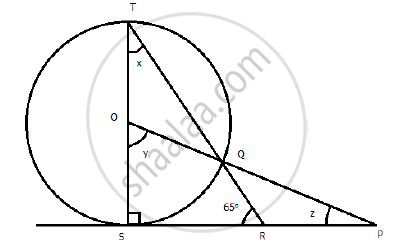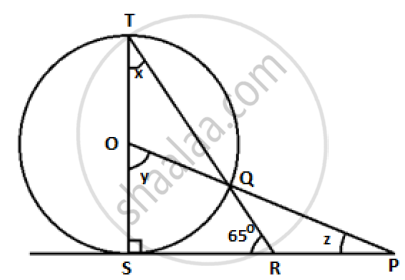Share

# In the Figure Given Below, O is the Centre of the Circle and Sp is a Tangent. If ∠Srt = 65°, Find the Value of X, Y and Z. - ICSE Class 10 - Mathematics

ConceptConstruction of Tangents to a Circle

#### Question

In the figure given below, O is the centre of the circle and SP is a tangent. If ∠SRT = 65°,
find the value of x, y and z.#### Solution

In the given figure, TS ⊥ SP,

m∠TSR = m∠OSP = 90°

In triangle TSR, m angleTSR + m angleTSR + m angle RTS = 180^@

=> 90^@ + 65^@ + x = 180^@

=> x = 180^@ - 90^@ - 65^@

=> x= 25^@

Now, y = 2x [Angle subtended at the centre is double that of the angle subtended by the arc at the same centre]

=> y = 2 xx 25^@

:. y = 50^@

In triangle OSP ,m angleOSP + m angle SPO + m angle POS    =180^@

=> 90^@ + z + 50^@ = 180^@

=> z = 180^@ - 140^@

:. z=40^@Is there an error in this question or solution?

#### APPEARS IN

2014-2015 (March) (with solutions)
Question 3.3 | 4.00 marks
Solution In the Figure Given Below, O is the Centre of the Circle and Sp is a Tangent. If ∠Srt = 65°, Find the Value of X, Y and Z. Concept: Construction of Tangents to a Circle.
S